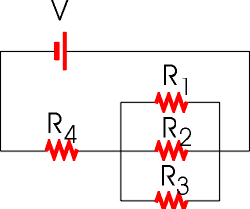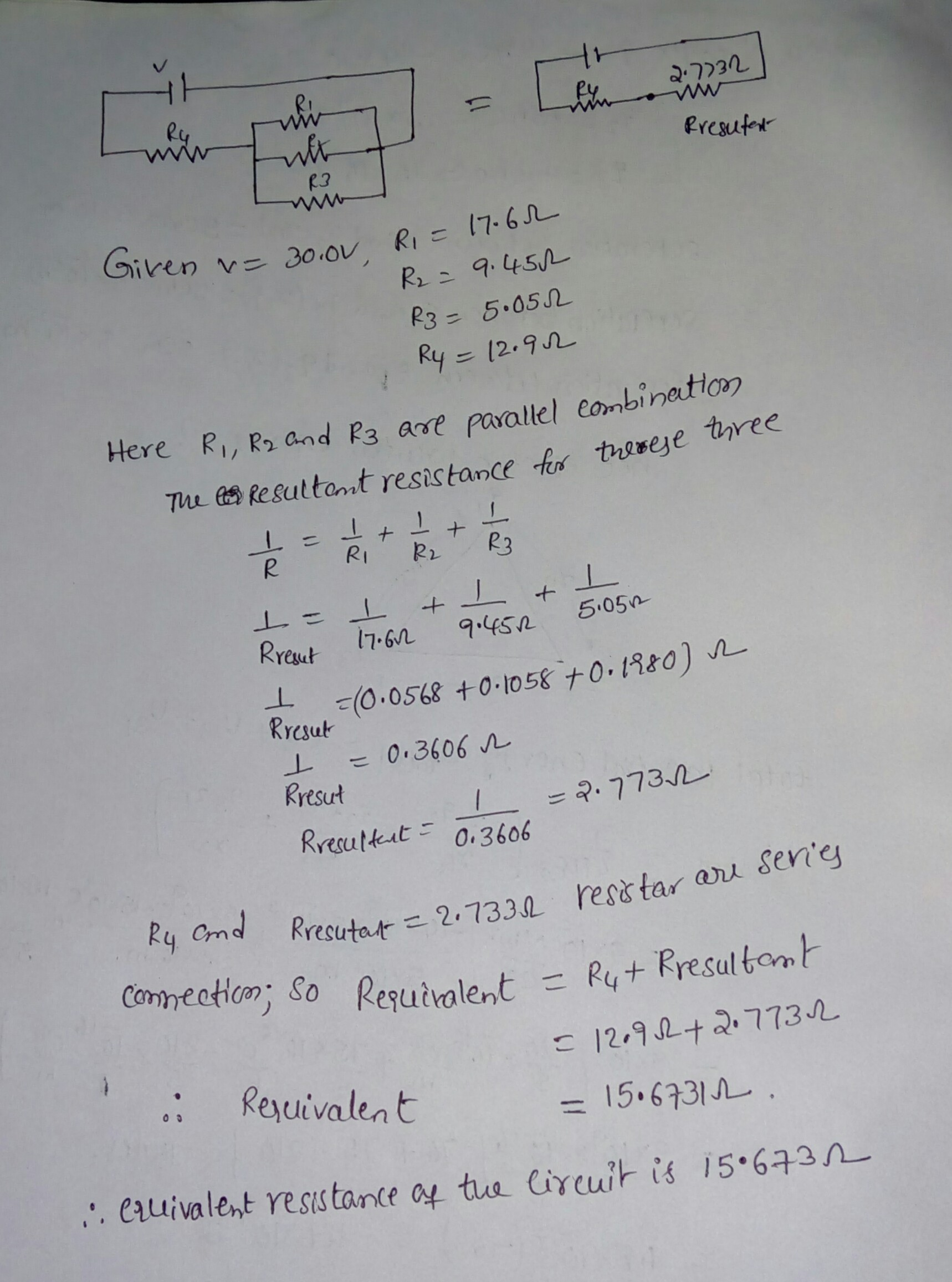# Find the equivalent resistance of the circuit shown in the figure.Find the equivalent resistance of the circuit shown in the
figure. Assume that V = 30.0 V, R1 = 17.6 ?, R2 = 9.45 ?, R3 = 5.05
? and R4 = 12.9 ?. RwRWR

Qo Presufet r3 Pg= 5.05 L Rys 12-9 Here Ri, P and Pa ave pavallel eonbination Tu &s Resuttat resistance for tree Rzesutr(0.0568 +0.1058ナ0-1980) n- Rresut Rreutkt 0,3606 ruto 2.733a restar au sen’e 129773 = 15-6731 Rexuivalent ‘. eruivalent resustante of tua Cirei is 15.633n